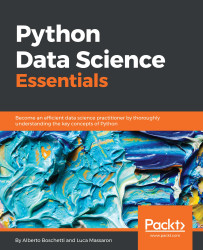•#### Python Data Science Essentials#### Overview of this book

The book starts by introducing you to setting up your essential data science toolbox. Then it will guide you across all the data munging and preprocessing phases. This will be done in a manner that explains all the core data science activities related to loading data, transforming and fixing it for analysis, as well as exploring and processing it. Finally, it will complete the overview by presenting you with the main machine learning algorithms, the graph analysis technicalities, and all the visualization instruments that can make your life easier in presenting your results. In this walkthrough, structured as a data science project, you will always be accompanied by clear code and simplified examples to help you understand the underlying mechanics and real-world datasets.
Python Data Science EssentialsCreditswww.PacktPub.comPrefaceFree Chapter
First StepsData MungingThe Data Science PipelineMachine LearningSocial Network AnalysisVisualizationIndex## Linear and logistic regression

Linear and logistic regressions are the two methods that can be used to linearly predict a target value and target class respectively. Let's start with an example of linear regression.

In this section, we will use the Boston dataset, which contains 506 samples, 13 features (all real numbers), and a (real) numerical target. We will divide our dataset into two sections by using a so-called train/test split cross-validation to test our methodology (in the example, 80 percent of our dataset goes in training, and 20 percent in the test):

```In: from sklearn.datasets import load_boston